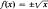# Single-Valued Function

Also found in: Dictionary, Thesaurus, Wikipedia.
Related to Single-Valued Function: Multivalued function

## single-valued function

[¦siŋ·gəl ‚val·yüd ′fəŋk·shən]
(mathematics)
A function for which exactly one point in the range corresponds to each point in the domain; a function that associates to each value of the independent variable exactly one value of the dependent variable. Also known as one-valued function.

## Single-Valued Function

a function that maps each value of the independent variable for which it is defined to just one value of the dependent variable—in contrast to a multiple-valued function. For example, f(x) = x2 is a single-valued function, whileis not because to each value of x other than zero there correspond two values of f(x), which differ in sign.

Site: Follow: Share:
Open / Close